# Part AHow many mmoles of Mg2+are present in 1 L of a Normosol-R solution that contains 4.3 mEq of Mg²+ ?Express your answer in mmoles to two significant figures.ΑΣφmmoles

Question
12 views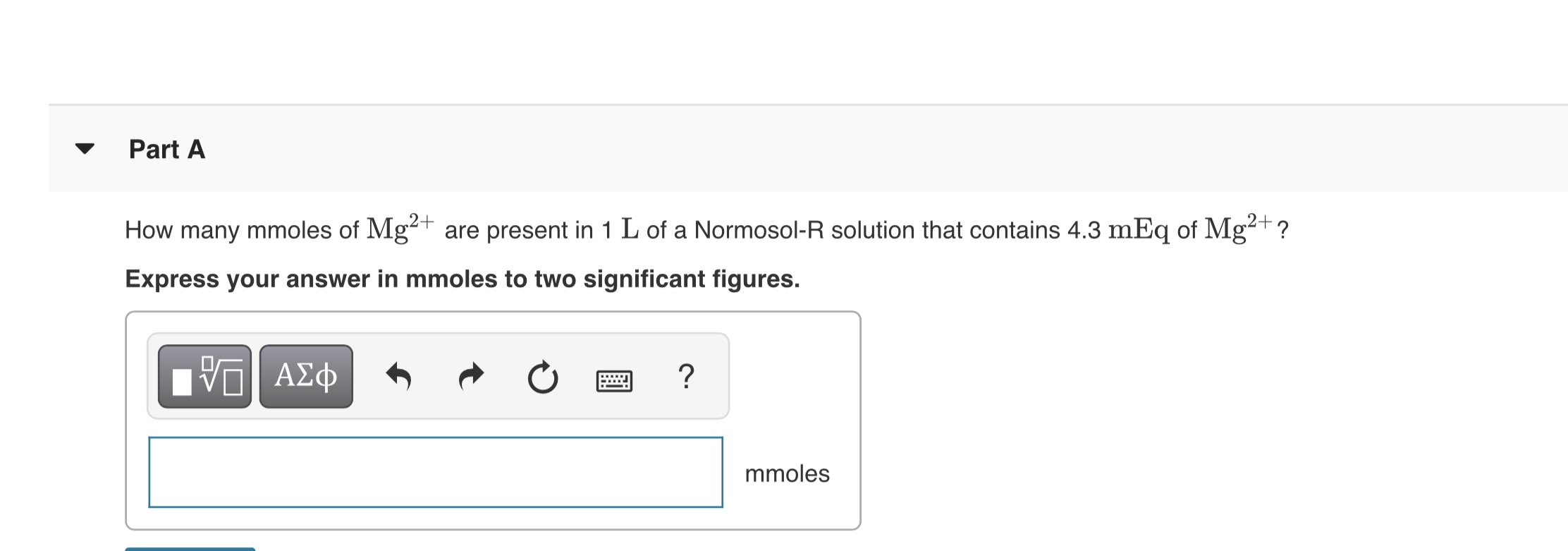help_outlineImage TranscriptionclosePart A How many mmoles of Mg2+ are present in 1 L of a Normosol-R solution that contains 4.3 mEq of Mg²+ ? Express your answer in mmoles to two significant figures. ΑΣφ mmoles fullscreen
check_circle

Introduction

The term "mole" provides the information about the substance's quantity. If the values of substance's molar mass (in g/mol) and mass (in g) are known, then one can evaluate the total moles of that specific substance.

Given Data:

The volume of solution is 1 L.

The equivalent mass of Mg2+ is 4.3 mEq.

Calculations

It is given that the solution contains 4.3 mEq of Mg2+.

Therefore,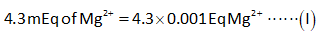The equivalent weight of Mg2+ ion is calculated by using the expression shown below.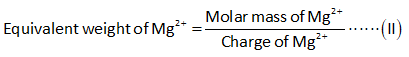The molar mass of Mg2+ ion is 24 g.

Substitute the known values in the above expression.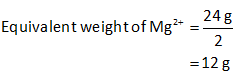Put the value of equivalent weight of Mg2+ in the expression (I).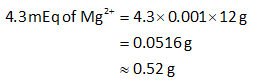Therefore, 1 L of solution contains 0.052 g of Mg2+.

Now, the number of moles of Mg2+ can be calculated as shown below.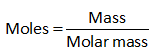Substitute the known values in the above expression.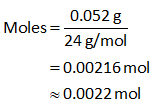The conversion of moles into milimoles can be done as follows: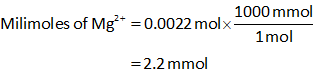...

### Want to see the full answer?

See Solution

#### Want to see this answer and more?

Solutions are written by subject experts who are available 24/7. Questions are typically answered within 1 hour.*

See Solution
*Response times may vary by subject and question.
Tagged in